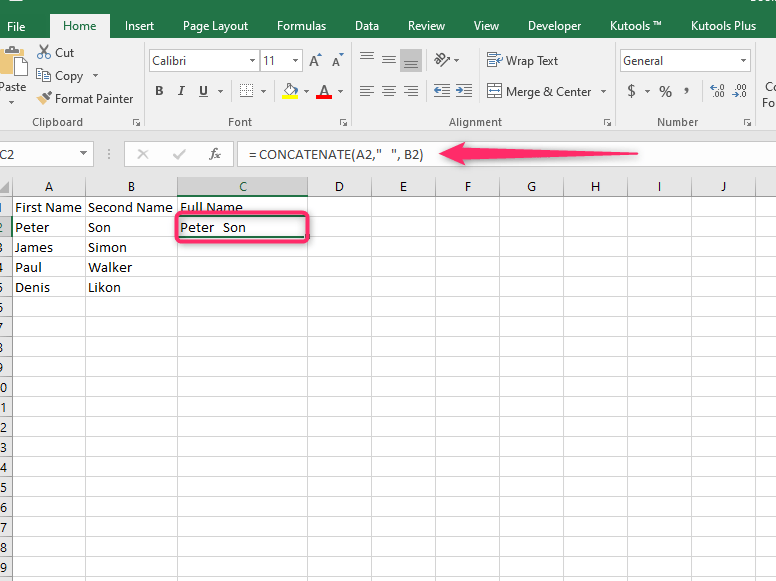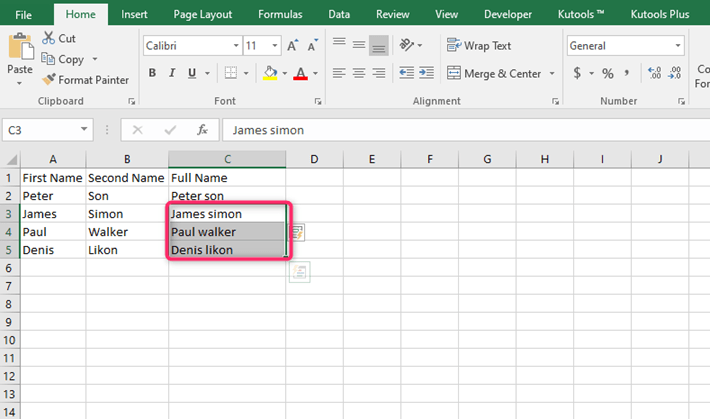# How to combine First and Second Name in Excel

Excel is a cell-based working platform, and therefore it is more convenient to place the first and the second name on each column. However, while working on this data, you may need to merge the first and the second name in one column. This may be possible using the following methods:

## Method 1: Using the Ampersand (&) symbol.

The ampersand symbol is greatly used in Excel to combine data found in two different cells and columns. It combines the two cells and returns the data in one cell.

Here are the steps to use this method:

1. Enter the first name on the first column and the second name on the second column.

2. Then, select a different column where the output will be stored. For example, name this column "Full Name."

3. Select a blank cell on the full name column, and enter the merging formula that contains the & symbol.

4. On the formula bar, type the equal symbol first. That is, =

5. Then, select the first cell that contains your first name to be merged. For example, =A2

6. Enter the ampersand symbol followed by the empty quotation marks. That is, =A2
& " "7. Then, select the second cell that contains the second name. That is, =A2 & " " B28. Finally, hit the enter button. Your first and second names will appear on the selected column.## Method 2: Using the Concatenation Formula

The concatenation formula can be used to merge two cells in different columns. Let us use the concatenation formula to combine the first and second names.

Steps:

1. Enter the first name on the first column and the second name on the second column.

2. Then, select a different column where the output will be stored. For example, name this column "Full Name."3. Select a blank cell on the full name column, and enter the merging formula that contains the & symbol.

4. On the formula bar, type the equal symbol followed by the keyword CONCATENATE, That is,
= CONCATENATE(

5. Then, select the first cell that contains your first name to be merged. For example, ,
= CONCATENATE(A2,

6. Enter space that will differentiate between the first and second names. For example, ,
= CONCATENATE(A2," ",7. Select the second cell that contains the second name, and terminate the formula with a closing bracket. For example, , = CONCATENATE(A2," ", B2)8. Finally, hit the enter button. Your first and second names will appear on the selected column.## Method 3: To combine automatically

Excel has a feature known as Flash fill that can automatically fill the data depending on the pattern. Flash fill can be used to combine the first and second name using these steps:

1. Enter the first and second names on a different column. Then select a different column where you'll store your results.

2. For the first cell, combine the column manually.

3. On the second cell, start typing, and excel will automatically suggest the full name.4. Press enters, and the two columns will combine automatically.